# 1.8 The real numbers  (Page 5/13)

 Page 5 / 13
$\begin{array}{ccccccc}\hfill \frac{7}{4}=1\frac{3}{4}\hfill & & & \hfill -\phantom{\rule{0.2em}{0ex}}\frac{9}{2}=-4\frac{1}{2}\hfill & & & \hfill \frac{8}{3}=2\frac{2}{3}\hfill \end{array}$

[link] shows the number line with all the points plotted.

Locate and label the following on a number line: $4,\frac{3}{4},-\phantom{\rule{0.2em}{0ex}}\frac{1}{4},-3,\frac{6}{5},-\phantom{\rule{0.2em}{0ex}}\frac{5}{2},\phantom{\rule{0.2em}{0ex}}\text{and}\phantom{\rule{0.2em}{0ex}}\frac{7}{3}.$

Locate and plot the integers, $4,-3.$

Locate the proper fraction $\frac{3}{4}$ first. The fraction $\frac{3}{4}$ is between 0 and 1. Divide the distance between 0 and 1 into four equal parts then, we plot $\frac{3}{4}.$ Similarly plot $-\phantom{\rule{0.2em}{0ex}}\frac{1}{4}.$

Now locate the improper fractions $\frac{6}{5},-\phantom{\rule{0.2em}{0ex}}\frac{5}{2},\frac{7}{3}.$ It is easier to plot them if we convert them to mixed numbers and then plot them as described above: $\frac{6}{5}=1\frac{1}{5},-\phantom{\rule{0.2em}{0ex}}\frac{5}{2}=-2\frac{1}{2},\frac{7}{3}=2\frac{1}{3}.$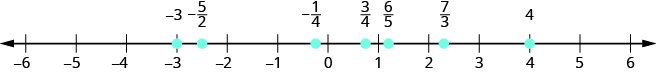Locate and label the following on a number line: $-1,\frac{1}{3},\frac{6}{5},-\phantom{\rule{0.2em}{0ex}}\frac{7}{4},\frac{9}{2},5,-\phantom{\rule{0.2em}{0ex}}\frac{8}{3}.$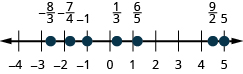Locate and label the following on a number line: $-2,\frac{2}{3},\frac{7}{5},-\phantom{\rule{0.2em}{0ex}}\frac{7}{4},\frac{7}{2},3,-\phantom{\rule{0.2em}{0ex}}\frac{7}{3}.$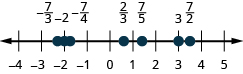In [link] , we’ll use the inequality symbols to order fractions. In previous chapters we used the number line to order numbers.

• a<b a is less than b ” when a is to the left of b on the number line
• a>b a is greater than b ” when a is to the right of b on the number line

As we move from left to right on a number line, the values increase.

Order each of the following pairs of numbers, using<or>. It may be helpful to refer [link] .

$-\phantom{\rule{0.2em}{0ex}}\frac{2}{3}___-1$ $-3\frac{1}{2}___-3$ $-\phantom{\rule{0.2em}{0ex}}\frac{3}{4}___-\phantom{\rule{0.2em}{0ex}}\frac{1}{4}$ $-2___-\phantom{\rule{0.2em}{0ex}}\frac{8}{3}$

Be careful when ordering negative numbers.

1. $\begin{array}{cccccc}& & & & & -\phantom{\rule{0.2em}{0ex}}\frac{2}{3}___-1\hfill \\ -\phantom{\rule{0.2em}{0ex}}\frac{2}{3}\phantom{\rule{0.2em}{0ex}}\text{is to the right of}\phantom{\rule{0.2em}{0ex}}-1\phantom{\rule{0.2em}{0ex}}\text{on the number line.}\hfill & & & & & -\phantom{\rule{0.2em}{0ex}}\frac{2}{3}>-1\hfill \end{array}$

2. $\begin{array}{cccccc}& & & & & -3\frac{1}{2}___-3\hfill \\ -3\frac{1}{2}\phantom{\rule{0.2em}{0ex}}\text{is to the left of}\phantom{\rule{0.2em}{0ex}}-3\phantom{\rule{0.2em}{0ex}}\text{on the number line.}\hfill & & & & & -3\frac{1}{2}<-3\hfill \end{array}$

3. $\begin{array}{cccccc}& & & & & -\phantom{\rule{0.2em}{0ex}}\frac{3}{4}___-\phantom{\rule{0.2em}{0ex}}\frac{1}{4}\hfill \\ -\phantom{\rule{0.2em}{0ex}}\frac{3}{4}\phantom{\rule{0.2em}{0ex}}\text{is to the left of}\phantom{\rule{0.2em}{0ex}}-\phantom{\rule{0.2em}{0ex}}\frac{1}{4}\phantom{\rule{0.2em}{0ex}}\text{on the number line.}\hfill & & & & & -\phantom{\rule{0.2em}{0ex}}\frac{3}{4}<-\phantom{\rule{0.2em}{0ex}}\frac{1}{4}\hfill \end{array}$

4. $\begin{array}{cccccc}& & & & & -2___-\phantom{\rule{0.2em}{0ex}}\frac{8}{3}\hfill \\ -2\phantom{\rule{0.2em}{0ex}}\text{is to the right of}\phantom{\rule{0.2em}{0ex}}-\phantom{\rule{0.2em}{0ex}}\frac{8}{3}\phantom{\rule{0.2em}{0ex}}\text{on the number line.}\hfill & & & & & -2>-\phantom{\rule{0.2em}{0ex}}\frac{8}{3}\hfill \end{array}$

Order each of the following pairs of numbers, using<or>:

$-\phantom{\rule{0.2em}{0ex}}\frac{1}{3}___-1$ $-1\frac{1}{2}___-2$ $-\phantom{\rule{0.2em}{0ex}}\frac{2}{3}___-\phantom{\rule{0.2em}{0ex}}\frac{1}{3}$ $-3___-\phantom{\rule{0.2em}{0ex}}\frac{7}{3}.$

> > < <

Order each of the following pairs of numbers, using<or>:

$-1___-\phantom{\rule{0.2em}{0ex}}\frac{2}{3}$ $-2\frac{1}{4}___-2$ $-\phantom{\rule{0.2em}{0ex}}\frac{3}{5}___-\phantom{\rule{0.2em}{0ex}}\frac{4}{5}$ $-4___-\phantom{\rule{0.2em}{0ex}}\frac{10}{3}.$

< < > <

## Locate decimals on the number line

Since decimals are forms of fractions, locating decimals on the number line is similar to locating fractions on the number line.

Locate 0.4 on the number line.

A proper fraction has value less than one. The decimal number 0.4 is equivalent to $\frac{4}{10},$ a proper fraction, so 0.4 is located between 0 and 1. On a number line, divide the interval between 0 and 1 into 10 equal parts. Now label the parts 0.1, 0.2, 0.3, 0.4, 0.5, 0.6, 0.7, 0.8, 0.9, 1.0. We write 0 as 0.0 and 1 and 1.0, so that the numbers are consistently in tenths. Finally, mark 0.4 on the number line. See [link] .

Locate on the number line: 0.6.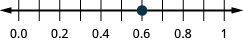Locate on the number line: 0.9.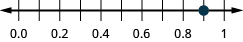Locate $-0.74$ on the number line.

The decimal $-0.74$ is equivalent to $-\phantom{\rule{0.2em}{0ex}}\frac{74}{100},$ so it is located between 0 and $-1.$ On a number line, mark off and label the hundredths in the interval between 0 and $-1.$ See [link] .

Locate on the number line: $-0.6.$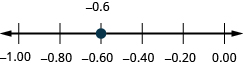Locate on the number line: $-0.7.$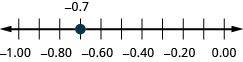Which is larger, 0.04 or 0.40? If you think of this as money, you know that $0.40 (forty cents) is greater than$0.04 (four cents). So,

$0.40>0.04$

Again, we can use the number line to order numbers.

• a<b a is less than b ” when a is to the left of b on the number line
• a>b a is greater than b ” when a is to the right of b on the number line

Where are 0.04 and 0.40 located on the number line? See [link] .

We see that 0.40 is to the right of 0.04 on the number line. This is another way to demonstrate that 0.40>0.04.

How does 0.31 compare to 0.308? This doesn’t translate into money to make it easy to compare. But if we convert 0.31 and 0.308 into fractions, we can tell which is larger.

Lucinda has a pocketful of dimes and quarters with a value of $6.20. The number of dimes is 18 more than 3 times the number of quarters. How many dimes and how many quarters does Lucinda have? Rhonda Reply Find an equation for the line that passes through the point P ( 0 , − 4 ) and has a slope 8/9 . Gabriel Reply is that a negative 4 or positive 4? Felix y = mx + b Felix if negative -4, then -4=8/9(0) + b Felix -4=b Felix if positive 4, then 4=b Felix then plug in y=8/9x - 4 or y=8/9x+4 Felix Macario is making 12 pounds of nut mixture with macadamia nuts and almonds. macadamia nuts cost$9 per pound and almonds cost $5.25 per pound. how many pounds of macadamia nuts and how many pounds of almonds should macario use for the mixture to cost$6.50 per pound to make?
Nga and Lauren bought a chest at a flea market for $50. They re-finished it and then added a 350 % mark - up Makaila Reply the sum of two Numbers is 19 and their difference is 15 Abdulai Reply 2, 17 Jose interesting saw Felecia left her home to visit her daughter, driving 45mph. Her husband waited for the dog sitter to arrive and left home 20 minutes, or 13 hour later. He drove 55mph to catch up to Felecia. How long before he reaches her? Rafi Reply integer greater than 2 and less than 12 Emily Reply 2 < x < 12 Felix I'm guessing you are doing inequalities... Felix Actually, translating words into algebraic expressions / equations... Felix hi Darianna hello Mister Eric here Eric He charges$125 per job. His monthly expenses are $1,600. How many jobs must he work in order to make a profit of at least$2,400?
at least 20
Ayla
what are the steps?
Alicia
6.4 jobs
Grahame
32
Grahame
1600+2400= total amount with expenses. 4000/125= number of jobs needed to make that min profit of 2400. answer is 32
Orlando
He must work 32 jobs to make a profit
POP
what is algebra
repeated addition and subtraction of the order of operations. i love algebra I'm obsessed.
Shemiah
hi
Krekar
Eric here. I'm a parent. 53 years old. I have never taken algebra. I want to learn.
Eric
One-fourth of the candies in a bag of M&M’s are red. If there are 23 red candies, how many candies are in the bag?
they are 92 candies in the bag
POP
rectangular field solutions
What is this?
Donna
t
muqtaar
the proudact of 3x^3-5×^2+3 and 2x^2+5x-4 in z7[x]/ is
?
Choli
a rock is thrown directly upward with an initial velocity of 96feet per second from a cliff 190 feet above a beach. The hight of tha rock above the beach after t second is given by the equation h=_16t^2+96t+190
Usman
Stella bought a dinette set on sale for $725. The original price was$1,299. To the nearest tenth of a percent, what was the rate of discount?
44.19%
Scott
40.22%
Terence
44.2%
Orlando
I don't know
Donna
if you want the discounted price subtract $725 from$1299. then divide the answer by $1299. you get 0.4419... but as percent you get 44.19... but to the nearest tenth... round .19 to .2 and you get 44.2% Orlando you could also just divide$725/\$1299 and then subtract it from 1. then you get the same answer.
Orlando
p mulripied-5 and add 30 to it
Tausif
Tausif
how
muqtaar
Can you explain further
p mulripied-5 and add to 30
Tausif
-5p+30?
Corey
p=-5+30
JacobBy Rachel CarlisleBy OpenStaxBy Stephen VoronBy OpenStaxBy Michael NelsonBy Richley CrapoBy Inderjeet BrarBy OpenStaxBy Brooke DelaneyBy Stephen Voron